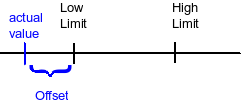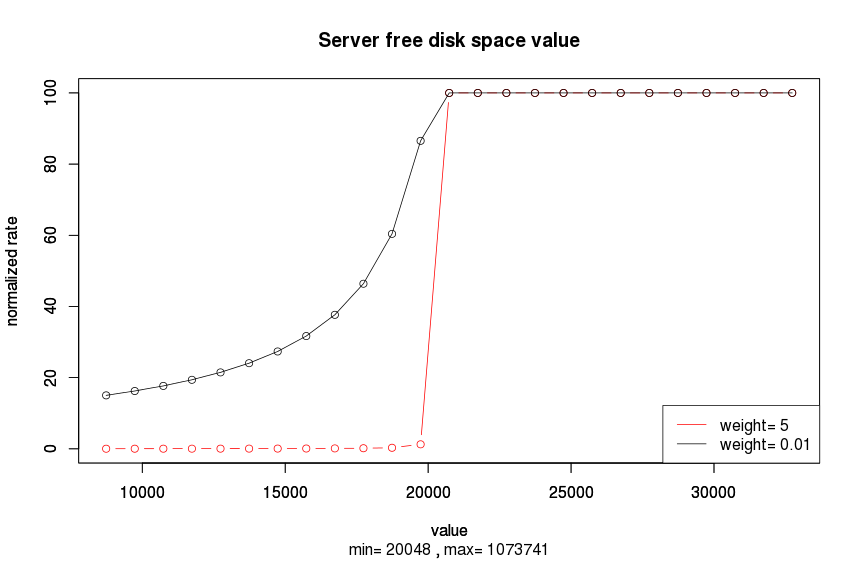# How to compute the Job server rate - 7.2

## Talend Data Services Platform Installation Guide for Windows

EnrichVersion
7.2
EnrichProdName
Talend Data Services Platform
EnrichPlatform
Talend Activity Monitoring Console
Talend Artifact Repository
Talend CommandLine
Talend Data Preparation
Talend Data Stewardship
Talend DQ Portal
Talend ESB
Talend Identity and Access Management
Talend Installer
Talend JobServer
Talend Log Server
Talend Repository Manager
Talend Runtime
Talend SAP RFC Server
Talend Studio

Let's define the offset of the feature as the difference between the limit and the actual value.Offset{i}= value{i} - Max{i}, when value{i} > Max{i} 0, when Min{i} < value{i} < Max{i} Min{i} - value{i}, when value{i} < Min{i}

The relative offset is given by the offset divided by the range:

``rel_offset{i} = offset{i} / [ Max{i} - Min{i} ]``

It's a positive value.

The rate of the server is computed as a weighted sum of all its relative offsets times a factor of -100:

``rate = -100 Σ weight{i} x rel_offset{i}``

At this stage, the rate is an unbounded negative number. In order to get a number between 0 and 100, the following formula is used:

``normalized rate = 100 / [ 1 - rate / scale ]``

where `scale = 2000`.

The scale is an arbitrary number that indicates the sensitivity to bad values. A normalized rate equal to 100 means that the server is good. A normalized rate lower than 100 means that the server is not so good. The lower the normalized rate is, the worst is the server. When all feature values are in the expected ranges, then the rate is 0 and the normalized rate is 100.

If the disk space is required to be between 1 GB and 2 GB and the actual disk space is 500 MB, then the relative offset is 1/2. The weight being 8, the normalized rate will be `100/ [ 1 + 400/2000 ] = 83.33`.

The below chart shows how the rate evolves according to the free disk space of the server and for two different weights (supposing that all other server features are inside the defined limits).The server rate updates each 90 seconds, which could be changed by setting the value of the parameter `notification.conf.checking.frequencyCheckJobServerState`.

Talend Administration Center lists rated servers in descending order, checks one by one from higher rate to lower rate to find out the best one, which should also have deployed Job.